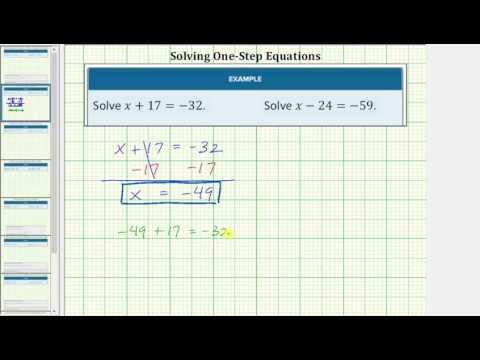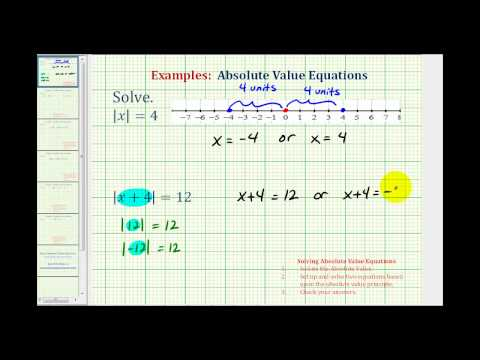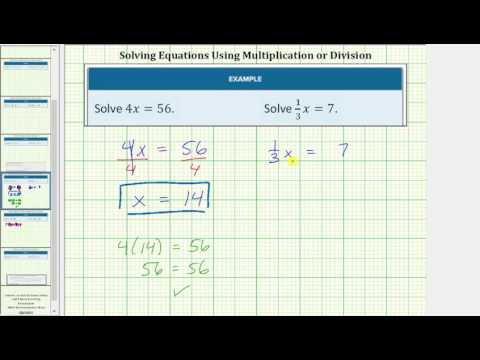$$\newcommand{\id}{\mathrm{id}}$$ $$\newcommand{\Span}{\mathrm{span}}$$ $$\newcommand{\kernel}{\mathrm{null}\,}$$ $$\newcommand{\range}{\mathrm{range}\,}$$ $$\newcommand{\RealPart}{\mathrm{Re}}$$ $$\newcommand{\ImaginaryPart}{\mathrm{Im}}$$ $$\newcommand{\Argument}{\mathrm{Arg}}$$ $$\newcommand{\norm}{\| #1 \|}$$ $$\newcommand{\inner}{\langle #1, #2 \rangle}$$ $$\newcommand{\Span}{\mathrm{span}}$$

# 1.2: Solving Linear Equations

$$\newcommand{\vecs}{\overset { \rightharpoonup} {\mathbf{#1}} }$$ $$\newcommand{\vecd}{\overset{-\!-\!\rightharpoonup}{\vphantom{a}\smash {#1}}}$$$$\newcommand{\id}{\mathrm{id}}$$ $$\newcommand{\Span}{\mathrm{span}}$$ $$\newcommand{\kernel}{\mathrm{null}\,}$$ $$\newcommand{\range}{\mathrm{range}\,}$$ $$\newcommand{\RealPart}{\mathrm{Re}}$$ $$\newcommand{\ImaginaryPart}{\mathrm{Im}}$$ $$\newcommand{\Argument}{\mathrm{Arg}}$$ $$\newcommand{\norm}{\| #1 \|}$$ $$\newcommand{\inner}{\langle #1, #2 \rangle}$$ $$\newcommand{\Span}{\mathrm{span}}$$ $$\newcommand{\id}{\mathrm{id}}$$ $$\newcommand{\Span}{\mathrm{span}}$$ $$\newcommand{\kernel}{\mathrm{null}\,}$$ $$\newcommand{\range}{\mathrm{range}\,}$$ $$\newcommand{\RealPart}{\mathrm{Re}}$$ $$\newcommand{\ImaginaryPart}{\mathrm{Im}}$$ $$\newcommand{\Argument}{\mathrm{Arg}}$$ $$\newcommand{\norm}{\| #1 \|}$$ $$\newcommand{\inner}{\langle #1, #2 \rangle}$$ $$\newcommand{\Span}{\mathrm{span}}$$

### Learning Objectives

• Use the addition property of equality
• Solve algebraic equations using the addition property of equality
• Solve one-step equations containing absolute values with addition
• Use the multiplication property of equality
• Solve algebraic equations using the multiplication property of equality
• Solve one-step equations containing absolute values with multiplication

## Solve an algebraic equation using the addition property of equality

First, let’s define some important terminology:

• variables: variables are symbols that stand for an unknown quantity, they are often represented with letters, like x, y, or z.
• coefficient: Sometimes a variable is multiplied by a number. This number is called the coefficient of the variable. For example, the coefficient of 3x is 3.
• term: a single number, or variables and numbers connected by multiplication. -4, 6x and $$x^2$$ are all terms
• expression: groups of terms connected by addition and subtraction. $$2x^2-5$$ is an expression
• equation: an equation is a mathematical statement that two expressions are equal. An equation will always contain an equal sign with an expression on each side. Think of an equal sign as meaning “the same as.” Some examples of equations are $$\frac{3}{4}r = v^{3} - r$$, and $$2(6-d) + f(3 +k) = \frac{1}{4}d$$

The following figure shows how coefficients, variables, terms, and expressions all come together to make equations. In the equation $$x$$, a coefficient is $$10x$$, an expression is $$2x-3^2$$.Equation made of coefficients, variables, terms and expressions.

### Using the Addition Property of Equality

An important property of equations is one that states that you can add the same quantity to both sides of an equation and still maintain an equivalent equation. Sometimes people refer to this as keeping the equation “balanced.” If you think of an equation as being like a balance scale, the quantities on each side of the equation are equal, or balanced.

Let’s look at a simple numeric equation, $$3+7=10$$, to explore the idea of an equation as being balanced.The expressions on each side of the equal sign are equal, so you can add the same value to each side and maintain the equality. Let’s see what happens when 5 is added to each side.

$$3+7+5=10+5$$

Since each expression is equal to 15, you can see that adding 5 to each side of the original equation resulted in a true equation. The equation is still “balanced.”

On the other hand, let’s look at what would happen if you added 5 to only one side of the equation.

$$\begin{array}{r}3+7=10\\3+7+5=10\\15\neq 10\end{array}$$

Adding 5 to only one side of the equation resulted in an equation that is false. The equation is no longer “balanced,” and it is no longer a true equation!

For all real numbers a, b, and c: If $$a+c=b+c$$.

If two expressions are equal to each other, and you add the same value to both sides of the equation, the equation will remain equal.

### Solve algebraic equations using the addition property of equality

When you solve an equation, you find the value of the variable that makes the equation true. In order to solve the equation, you isolate the variable. Isolating the variable means rewriting an equivalent equation in which the variable is on one side of the equation and everything else is on the other side of the equation.

When the equation involves addition or subtraction, use the inverse operation to “undo” the operation in order to isolate the variable. For addition and subtraction, your goal is to change any value being added or subtracted to 0, the additive identity.

In the following simulation, you can adjust the quantity being added or subtracted to each side of an equation to see how important it is to perform the same operation on both sides of an equation when you are solving.

### Examples

Solve $$x-6=8$$.

This equation means that if you begin with some unknown number, x, and subtract 6, you will end up with 8. You are trying to figure out the value of the variable x.

Using the Addition Property of Equality, add 6 to both sides of the equation to isolate the variable. You choose to add 6 because 6 is being subtracted from the variable.

$$\displaystyle \begin{array}{r}x-6\,\,\,=\,\,\,\,8\\\,\,\,\,\,\,\,\underline{+\,6\,\,\,\,\,\,\,\,+6}\\\,\,\,\,\,\,\,\,\,\,\,\,\,x\,\,=\, 14\end{array}$$

$$x=14$$

Solve $$x+5=27$$.

This equation means that if you begin with some unknown number, x, and add 5, you will end up with 27. You are trying to figure out the value of the variable x.

Using the Addition Property of Equality, subtract 5 from both sides of the equation to isolate the variable. You choose to subtract 5, as 5 is being added from the variable.

$$\displaystyle \begin{array}{r}x+5\,\,=\,\,27\\\,\,\,\,\,\,\,\underline{-5\,\,\,\,\,\,\,\,-5}\\\,\,\,\,\,\,\,\,\,\,\,\,\,x\,\,=\, 22\end{array}$$

$$x=22$$

In the following video two examples of using the addition property of equality are shown.A YouTube element has been excluded from this version of the text. You can view it online here: pb.libretexts.org/ba/?p=46

Since subtraction can be written as addition (adding the opposite), the addition property of equality can be used for subtraction as well. So just as you can add the same value to each side of an equation without changing the meaning of the equation, you can subtract the same value from each side of an equation.

### Examples

Solve $$x+10=-65$$. Check your solution.

Solve:

$$x+10=-65$$

Since 10 is being added to the variable, subtract 10 from both sides. Note that subtracting 10 is the same as adding $$–10$$.

$$\displaystyle \begin{array}{r}x+10\,\,=\,\,\,\,-65\\\,\,\,\,\,\underline{-10\,\,\,\,\,\,\,\,\,\,-10}\\\,\,\,\,\,\,\,\,\,\,\,x\,\,\,\,=\,\,\,-75\end{array}$$

To check, substitute the solution, $$–75$$ for x in the original equation, then simplify.

$$\displaystyle \begin{array}{r}\,\,\,\,\,x+10\,\,\,=-65\\-75+\,10\,\,\,=-65\\\,\,\,\,\,\,\,\,\,\,\,-65\,\,\,=-65\end{array}$$

This equation is true, so the solution is correct.

$$x+10=–65$$.

Solve $$x-4=-32$$. Check your solution.

Solve:

$$x-4=-32$$

Since 4 is being subtracted from the variable, add 4 to both sides.

$$\displaystyle \begin{array}{r}x-4\,\,=\,\,\,\,-32\\\,\,\,\,\,\underline{+4\,\,\,\,\,\,\,\,\,\,+4}\\\,\,\,\,\,\,\,\,\,\,\,x\,\,\,\,=\,\,\,-28\end{array}$$

Check:

To check, substitute the solution, $$–28$$ for x in the original equation, then simplify.

$$\displaystyle \begin{array}{r}\,\,\,\,\,x-4\,\,\,=-32\\-28-\,4\,\,\,=-32\\\,\,\,\,\,\,\,\,\,\,\,-32\,\,\,=-32\end{array}$$

This equation is true, so the solution is correct.

$$x-4=–32$$.

It is always a good idea to check your answer whether you are requested to or not.

The following video presents two examples of using the addition property of equality when there are negative integers in the equation.A YouTube element has been excluded from this version of the text. You can view it online here: pb.libretexts.org/ba/?p=46

Can you determine what you would do differently if you were asked to solve equations like these?

a) Solve $${12.5}+{ t }= {-7.5}$$.

What makes this example different than the previous ones? Use the box below to write down a few thoughts about how you would solve this equation with decimals.

[practice-area rows=”2″][/practice-area]

[hidden-answer a=”680980″]To solve this equation you need to remember how to add or subtract decimal numbers. You also need to remember that when you subtract a number from a negative number, your result will be negative.

Using the Addition Property of Equality, subtract 12.5 from both sides of the equation to isolate the variable, t. You choose to subtract 12.5 because 12.5 is being added to the variable, t.

$$\displaystyle \begin{array}{r}{12.5}+{t}\,\,\,=\,\,\,\,{-7.5}\\\,\,\,\,\,\,\,\underline{-12.5\,\,\,\,\,\,\,\,-12.5}\\\,\,\,\,\,\,\,\,\,\,\,\,\,t\,\,=\, -20\end{array}$$

To add two numbers of the same sign, first add their absolute values:

$$\begin{array}{r}\left|-12.5\right| = 12.5\\\left|-7.5\right| = 7.5\,\,\,\\12.5 + 7.5 = 20\,\,\,\,\end{array}$$

Now apply the sign they share, which is negative:

$$-12.5 -7.5 = -20$$

b) Solve $$\frac{1}{4} + y = 3$$. What makes this example different than the previous ones? Use the box below to write down a few thoughts about how you would solve this equation with a fraction.

[practice-area rows=”2″][/practice-area]

Using the Addition Property of Equality, subtract $$\frac{1}{4}$$ as $$\frac{1}{4}$$ is being added to the variable, y.

$$\displaystyle\begin{array}{r}\frac{1}{4} + y\,\,\,=\,\,\,\,{3}\,\,\\\,\,\,\,\,\,\,\underline{-\frac{1}{4}\,\,\,\,\,\,\,\,\,\,\,\,\,\,\,\,-\frac{1}{4}}\\\,\,\,\,\,\,\,\,\,\,\,\,\,y\,\,=\,3-\frac{1}{4}\,\,\end{array}$$

To subtract $$\frac{1}{4}$$ from 3, you need a common denominator.

Make 3 into a fraction by dividing by 1, $$\frac{3}{1}$$. Your denominators are 1 and 4. The least common multiple is 4.

$$\begin{array}{r}\frac{3}{1}\cdot\frac{4}{4}=\frac{12}{4}\\\frac{12}{4} -\frac{1}{4} =\frac{11}{4}\end{array}$$

Therefore, $$y =3 -\frac{1}{4} =\frac{11}{4}$$.

In the following video, two examples of using the addition property of equality with decimal numbers are shown.A YouTube element has been excluded from this version of the text. You can view it online here: pb.libretexts.org/ba/?p=46

The next video shows how to use the addition property of equality to solve equations with fractions.A YouTube element has been excluded from this version of the text. You can view it online here: pb.libretexts.org/ba/?p=46

The examples above are sometimes called one-step equations because they require only one step to solve. In these examples, you either added or subtracted a constant from both sides of the equation to isolate the variable and solve the equation.

With any equation, you can check your solution by substituting the value for the variable in the original equation. In other words, you evaluate the original equation using your solution. If you get a true statement, then your solution is correct.

Writing and solving algebraic equations is an important part of mathematics. Equations can be used to describe economic, cultural, physical, and biological processes. They help business people make decisions, and help doctors and scientists find ways to heal and help people. Without mathematical equations we would not have the physical infrastructure that rely on every day for transportation and clean water.

Equations can help you model situations and solve problems in which quantities are unknown (like how long Joan should wait before she drives home). The simplest type of algebraic equation is a linear equation that has just one variable.

When you follow the steps to solve an equation, you try to isolate the variable. The variable is a quantity we don’t know yet. You have a solution when you get the equation x = some value.

## Solving One-Step Equations Containing Absolute Values with Addition

The absolute value of a number or expression describes its distance from 0 on a number line. Since the absolute value expresses only the distance, not the direction of the number on a number line, it is always expressed as a positive number or 0.

For example, $$−4$$ and 4 both have an absolute value of 4 because they are each 4 units from 0 on a number line—though they are located in opposite directions from 0 on the number line.

When solving absolute value equations and inequalities, you have to consider both the behavior of absolute value and the properties of equality and inequality.

Because both positive and negative values have a positive absolute value, solving absolute value equations means finding the solution for both the positive and the negative values.

Let’s first look at a very basic example.

$$\displaystyle \left| x \right|=5$$

This equation is read “the absolute value of x is equal to five.” The solution is the value(s) that are five units away from 0 on a number line.

You might think of 5 right away; that is one solution to the equation. Notice that $$−5$$ is 5 units away from 0 in the opposite direction. So, the solution to this equation $$x = −5$$ or $$x = 5$$.

### Solving Equations of the Form $$|x|=a$$

For any positive number a, the solution of $$\left|x\right|=a$$ is

$$x=−a$$

x can be a single variable or any algebraic expression.

You can solve a more complex absolute value problem in a similar fashion.

### Example

Solve $$\displaystyle \left| x+5\right|=15$$.

This equation asks you to find what number plus 5 has an absolute value of 15. Since 15 and $$x + 5$$ is 15 or $$−15$$, since $$|−15|=15$$. So, you need to find out what value for x will make this expression equal to 15 as well as what value for x will make the expression equal to $$−15$$. Solving the two equations you get

$$\displaystyle \begin{array}{l}x+5=15\,\,\,\,\,\,\,\text{or}\,\,\,\,\,\,\,{x+5=-15}\\\underline{\,\,\,\,\,-5\,\,\,\,-5}\,\,\,\,\,\,\,\,\,\,\,\,\,\,\,\,\,\,\,\,\,\,\,\underline{-5\,\,\,\,\,\,\,\,\,-5}\\x\,\,\,\,\,\,\,\,\,=\,\,10\,\,\,\,\,\,\,\text{or}\,\,\,\,\,\,\,x\,\,\,\,\,\,\,\,=-20\end{array}$$

You can check these two solutions in the absolute value equation to see if $$x=−20$$ are correct.

$$\displaystyle \begin{array}{r}\,\,\left| x+5 \right|=15\,\,\,\,\,\,\,\,\,\,\,\,\,\,\,\,\,\,\,\,\,\,\,\left| x+5 \right|=15\\\left| 10+5 \right|=15\,\,\,\,\,\,\,\,\,\,\,\,\,\,\,\,\left| -20+5 \right|=15\\\,\,\,\,\,\,\,\left| 15 \right|=15\,\,\,\,\,\,\,\,\,\,\,\,\,\,\,\,\,\,\,\,\,\,\,\,\,\,\left| -15 \right|=15\\\,\,\,\,\,\,\,\,\,15=15\,\,\,\,\,\,\,\,\,\,\,\,\,\,\,\,\,\,\,\,\,\,\,\,\,\,\,\,\,\,\,\,\,\,\,15=15\end{array}$$[/hidden-answer]

The following video provides worked examples of solving linear equations with absolute value terms.A YouTube element has been excluded from this version of the text. You can view it online here: pb.libretexts.org/ba/?p=46

## Solve algebraic equations using the multiplication property of equality

Just as you can add or subtract the same exact quantity on both sides of an equation, you can also multiply both sides of an equation by the same quantity to write an equivalent equation. Let’s look at a numeric equation, $$5\cdot3=15$$, to start. If you multiply both sides of this equation by 2, you will still have a true equation.

$$\begin{array}{r}5\cdot 3=15\,\,\,\,\,\,\, \\ 5\cdot3\cdot2=15\cdot2 \\ 30=30\,\,\,\,\,\,\,\end{array}$$

This characteristic of equations is generalized in the multiplication property of equality.

### Multiplication Property of Equality

For all real numbers a, b, and c: If a = b, then $$a\cdot{c}=b\cdot{c}$$ (or ab = ac).

If two expressions are equal to each other and you multiply both sides by the same number, the resulting expressions will also be equivalent.

When the equation involves multiplication or division, you can “undo” these operations by using the inverse operation to isolate the variable. When the operation is multiplication or division, your goal is to change the coefficient to 1, the multiplicative identity.

### Example

Solve $$3x=24$$. When you are done, check your solution.

[hidden-answer a=”42404″]Divide both sides of the equation by 3 to isolate the variable (have a coefficient of 1). Dividing by 3 is the same as having multiplied by $$\frac{1}{3}$$.

$$\begin{array}{r}\underline{3x}=\underline{24}\\3\,\,\,\,\,\,\,\,\,\,\,\,3\,\\x=8\,\,\,\end{array}$$

Check by substituting your solution, 8, for the variable in the original equation.

$$\begin{array}{r}3x=24 \\ 3\cdot8=24 \\ 24=24\end{array}$$

The solution is correct!

$$x=8$$

You can also multiply the coefficient by the multiplicative inverse (reciprocal) in order to change the coefficient to 1.

### Example

Solve $$\frac{1}{2}$$, which is 2.

Multiply both sides by 2:

$$\begin{array}{r}\left(2\right)\frac{1}{2 }{ x }=\left(2\right){ 8}\\\left(2\right)\frac{1}{2 } = 1\,\,\,\,\,\,\,\,\\\left(2\right)8 = 16\,\,\,\,\,\\{ x }=16\,\,\,\,\,\end{array}$$

In the video below you will see examples of how to use the multiplication property of equality to solve one-step equations with integers and fractions.A YouTube element has been excluded from this version of the text. You can view it online here: pb.libretexts.org/ba/?p=46

In the next example, we will solve a one-step equation using the multiplication property of equality. You will see that the variable is part of a fraction in the given equation, and using the multiplication property of equality allows us to remove the variable from the fraction. Remember that fractions imply division, so you can think of this as the variable k is being divided by 10. To “undo” the division, you can use multiplication to isolate k. Lastly, note that there is a negative term in the equation, so it will be important to think about the sign of each term as you work through the problem. Stop after each step you take to make sure all the terms have the correct sign.

### Example

Solve $$-\frac{7}{2}=\frac{k}{10}$$ for k.

[hidden-answer a=”471772″]We want to isolate the k, which is being divided by 10. The first thing we should do is multiply both sides by 10.

$$\begin{array}{l}\text{ multiply the left side by 10 }\\\\\left(10\right)-\frac{7}{2}=\frac{10\cdot7}{2} = \frac{70}{2} = 35\\\\\text{ now multiply the right side by 10 }\\\\\,\,\,\frac{k}{10}\left(10\right) = \frac{k\cdot10}{10} = k\\\\\text{ now replace your results in the equation }\\35=k\end{array}$$

We write the k on the left side as a matter of convention.

$$k=35$$

In the following video you will see examples of using the multiplication property of equality to solve a one-step equation involving negative fractions.A YouTube element has been excluded from this version of the text. You can view it online here: pb.libretexts.org/ba/?p=46

## Solving One-Step Equations Containing Absolute Values With Multiplication

In the last section, we saw examples of solving equations with absolute values where the only operation was addition or subtraction. Now we will see how to solve equations with absolute value that include multiplication.

Remember that absolute value refers to the distance from zero. You can use the same technique of first isolating the absolute value, then setting up and solving two equations to solve an absolute value equation involving multiplication.

### Example

Solve $$\displaystyle \left| 2x\right|=6$$.

This equation asks you to find what number times 2 has an absolute value of 6.

Since 6 and $$2x$$ is 6 or $$−6$$, since $$|−6|=6$$.

So, you need to find out what value for x will make this expression equal to 6 as well as what value for x will make the expression equal to $$−6$$.

Solving the two equations you get

$$2x=6\text{ or }2x=-6$$

$$\frac{2x}{2}=\frac{6}{2}\text{ or }\frac{2x}{2}=\frac{-6}{2}$$

$$x=3\text{ or }x=-3$$

You can check these two solutions in the absolute value equation to see if $$x=−3$$ are correct.

$$\displaystyle \begin{array}{r}\,\,\left|3\cdot2 \right|=6\,\,\,\,\,\,\,\,\,\,\,\,\,\,\,\,\,\,\left| -3\cdot2 \right|=6\\\left| 6 \right|=6\,\,\,\,\,\,\,\,\,\,\,\,\,\,\,\,\,\,\,\,\,\,\,\,\,\left| -6 \right|=6\\\end{array}$$[/hidden-answer]

### Example

Solve $$\displaystyle\frac{1}{3}\left|k\right|=12$$.

Notice how this example is different from the last; $$\displaystyle\frac{1}{3}$$ is outside the absolute value grouping symbols. This means we need to isolate the absolute value first, then apply the definition of absolute value.

First, isolate the absolute value term by multiplying by the inverse of $$\displaystyle\frac{1}{3}$$:

$$\begin{array}{r}\frac{1}{3}\left|k\right|=12\,\,\,\,\,\,\,\,\\\left(3\right)\frac{1}{3}\left|k\right|=\left(3\right)12\\\left|k\right|=36\,\,\,\,\,\,\,\,\end{array}$$

Apply the definition of absolute value:

$$\displaystyle{k }=36\text{ or }{k }=-36$$

You can check these two solutions in the absolute value equation to see if $$x=−36$$ are correct.

$$\displaystyle \begin{array}{r}\,\,\frac{1}{3}\left|36 \right|=12\,\,\,\,\,\,\,\,\,\,\,\,\,\,\,\,\,\,\frac{1}{3}\left|-36 \right|=12\\\left| 12 \right|=12\,\,\,\,\,\,\,\,\,\,\,\,\,\,\,\,\,\,\,\,\,\,\left| -12 \right|=12\\\end{array}$$[/hidden-answer]

In the following video you will see two examples of how to solve an absolute value equation, one with integers and one with fractions.A YouTube element has been excluded from this version of the text. You can view it online here: pb.libretexts.org/ba/?p=46